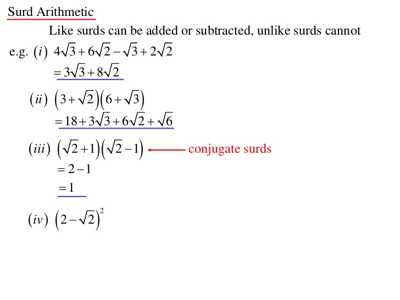Conjugate Surds

The sum and difference of two simple quadratic surds are said to be conjugate surds to each other.

Conjugate surds are also known as complementary surds. it can be used to express a fraction which has a compound surd as its denominator with a rational denominator. You multiply the top and bottom of the fraction by the conjugate of the bottom line.

Thus, the sum and the difference of two simple quadratic surds 4√7and √2 are 4√7 + √2 and 4√7 – √2   respectively. Therefore, two surds (4√7 + √2) and (4√7 – √2) are conjugate to each other.

Similarly, two surds (-2√5 + √3) and (-2√5 – √3) are conjugate to each other.

In general, two binomial quadratic surds (x√a + y√b) and (x√a – y√b) are conjugate to each other.The fundamental algebraic identities lead us to find the definition of conjugate surds. A square root of any positive number when multiplied by itself gives the product as the number inside the square root and hence, the product now becomes a rational number. That is, when b√b multiplied by b√b, the product is ‘b’ which is a rational number.

Similarly, when sum of two terms and the difference of the same two terms are multiplied, the product is always the difference of the squares of the terms. Let us apply this concept to a binomial surd (a + b√b). When we multiply this with the difference of the same two terms, that is, with (a – b√b), the product is (a)2 – (b√b)2 = a2 – b, which now becomes a rational number. The multipliers b√b in case of monomial surds and (a – b√b) in case of binomial surds are called as conjugate surds to the given surds.

Note:

1. Since 3 + √5 = √9 + √5 and surd conjugate to √9 + √5 is √9 – √5, hence it is evident that surds 3 + √5 and 3 – √5 are conjugate to each other.

In general, surds (a + x√b) and (a – x√b) are complementary to each other.

1. The product of two binomial quadratic surds is always rational.

For example,

(√m + √n)(√m – √n) = (√m)^2 – (√n)^2 = m – n, which is rational.

Information Source;